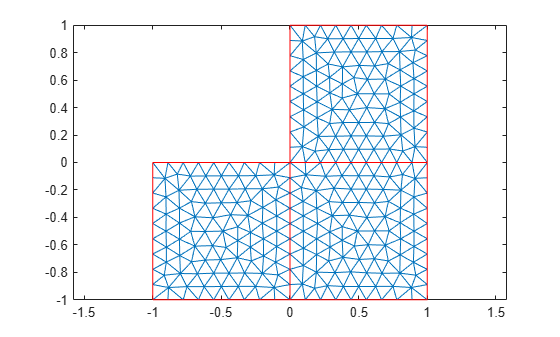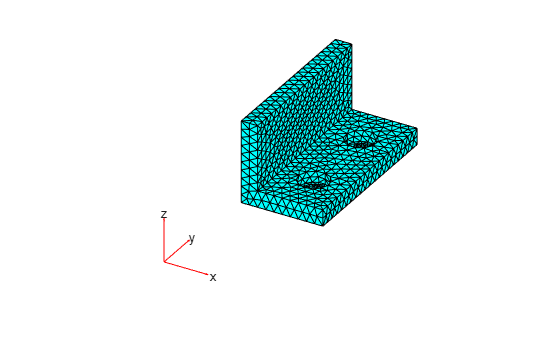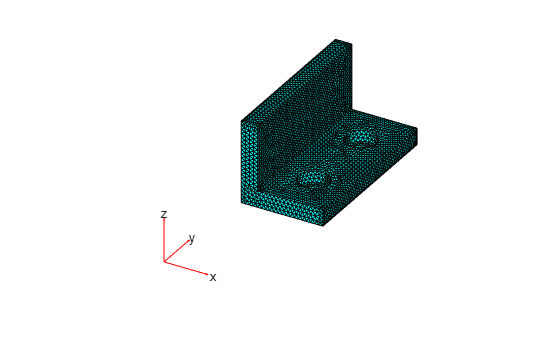# generateMesh

Create triangular or tetrahedral mesh

## Syntax

``generateMesh(model)``
``generateMesh(model,Name,Value)``
``mesh = generateMesh(___)``

## Description

example

````generateMesh(model)` creates a mesh and stores it in the `model` object. `model` must contain a geometry. To include 2-D geometry in a model, use `geometryFromEdges`. To include 3-D geometry, use `importGeometry` or `geometryFromMesh`.`generateMesh` can return slightly different meshes in different releases. For example, the number of elements in the mesh can change. Avoid writing code that relies on explicitly specified node and element IDs.```

example

````generateMesh(model,Name,Value)` modifies the mesh creation according to the `Name,Value` arguments.```
````mesh = generateMesh(___)` also returns the mesh to the MATLAB® workspace, using any of the previous syntaxes.```

## Examples

collapse all

Generate the default 2-D mesh for the L-shaped geometry.

Create a PDE model and include the L-shaped geometry.

```model = createpde(1); geometryFromEdges(model,@lshapeg);```

Generate the default mesh for the geometry.

`generateMesh(model);`

View the mesh.

`pdeplot(model)`Create a mesh that is finer than the default.

Create a PDE model and include the `BracketTwoHoles` geometry.

```model = createpde(1); importGeometry(model,'BracketTwoHoles.stl');```

Generate a default mesh for comparison.

`generateMesh(model)`
```ans = FEMesh with properties: Nodes: [3x10003 double] Elements: [10x5774 double] MaxElementSize: 9.7980 MinElementSize: 4.8990 MeshGradation: 1.5000 GeometricOrder: 'quadratic' ```

View the mesh.

`pdeplot3D(model)`Create a mesh with target maximum element size 5 instead of the default 7.3485.

`generateMesh(model,'Hmax',5)`
```ans = FEMesh with properties: Nodes: [3x66982 double] Elements: [10x44093 double] MaxElementSize: 5 MinElementSize: 2.5000 MeshGradation: 1.5000 GeometricOrder: 'quadratic' ```

View the mesh.

`pdeplot3D(model)`## Input Arguments

collapse all

Model object, specified as a `PDEModel` object, `ThermalModel` object, `StructuralModel` object, or `ElectromagneticModel` object.

Example: `model = createpde(3)`

Example: `thermalmodel = createpde('thermal','steadystate')`

Example: `structuralmodel = createpde('structural','static-solid')`

Example: ```emagmodel = createpde('electromagnetic','electrostatic')```

### Name-Value Pair Arguments

Specify optional comma-separated pairs of `Name,Value` arguments. `Name` is the argument name and `Value` is the corresponding value. `Name` must appear inside quotes. You can specify several name and value pair arguments in any order as `Name1,Value1,...,NameN,ValueN`.

Example: `generateMesh(model,'Hmax',0.25);`

Element geometric order, specified as the comma-separated pair consisting of `'GeometricOrder'` and `'linear'` or `'quadratic'`.

A triangle or tetrahedron representing a linear element has nodes at the corners. A triangle or tetrahedron representing a quadratic element has nodes at its corners and edge centers. The center nodes in quadratic meshes are always added at half-distance between corners. For geometries with curved surfaces and edges, center nodes might not appear on the edge or surface itself.

In general, `'quadratic'` elements produce more accurate solutions. Override the default `'quadratic'` only to save memory or to solve a 2-D problem using a legacy solver. Legacy PDE solvers use linear triangular mesh for 2-D geometries.

Example: `generateMesh(model,'GeometricOrder','linear');`

Data Types: `char` | `string`

Mesh growth rate, specified as the comma-separated pair consisting of `Hgrad` and a number greater than or equal to 1 and less than or equal to 2.

Example: `generateMesh(model,'Hgrad',1.3);`

Data Types: `double`

Target maximum mesh edge length, specified as the comma-separated pair consisting of `Hmax` and a positive real number.

`Hmax` is an approximate upper bound on the mesh edge lengths. Occasionally, `generateMesh` can create a mesh with some elements that exceed `Hmax`.

`generateMesh` estimates the default value of `Hmax` from overall dimensions of the geometry.

Small `Hmax` values let you create finer meshes, but mesh generation can take a very long time in this case. You can interrupt mesh generation by using Ctrl+C. Note that `generateMesh` can take additional time to respond to the interrupt.

Example: `generateMesh(model,'Hmax',0.25);`

Data Types: `double`

Target minimum mesh edge length, specified as the comma-separated pair consisting of `Hmin` and a nonnegative real number.

`Hmin` is an approximate lower bound on the mesh edge lengths. Occasionally, `generateMesh` can create a mesh with some elements that are smaller than `Hmin`.

`generateMesh` estimates the default value of `Hmin` from overall dimensions of the geometry.

Example: `generateMesh(model,'Hmin',0.05);`

Data Types: `double`

## Output Arguments

collapse all

Mesh description, returned as an FEMesh Properties object. `mesh` is the same as `model.Mesh`.

collapse all

### Element

An element is a basic unit in the finite-element method.

For 2-D problems, an element is a triangle in the `model.Mesh.Element` property. If the triangle represents a linear element, it has nodes only at the triangle corners. If the triangle represents a quadratic element, then it has nodes at the triangle corners and edge centers.

For 3-D problems, an element is a tetrahedron with either four or ten points. A four-point (linear) tetrahedron has nodes only at its corners. A ten-point (quadratic) tetrahedron has nodes at its corners and at the center point of each edge.

For details, see Mesh Data.